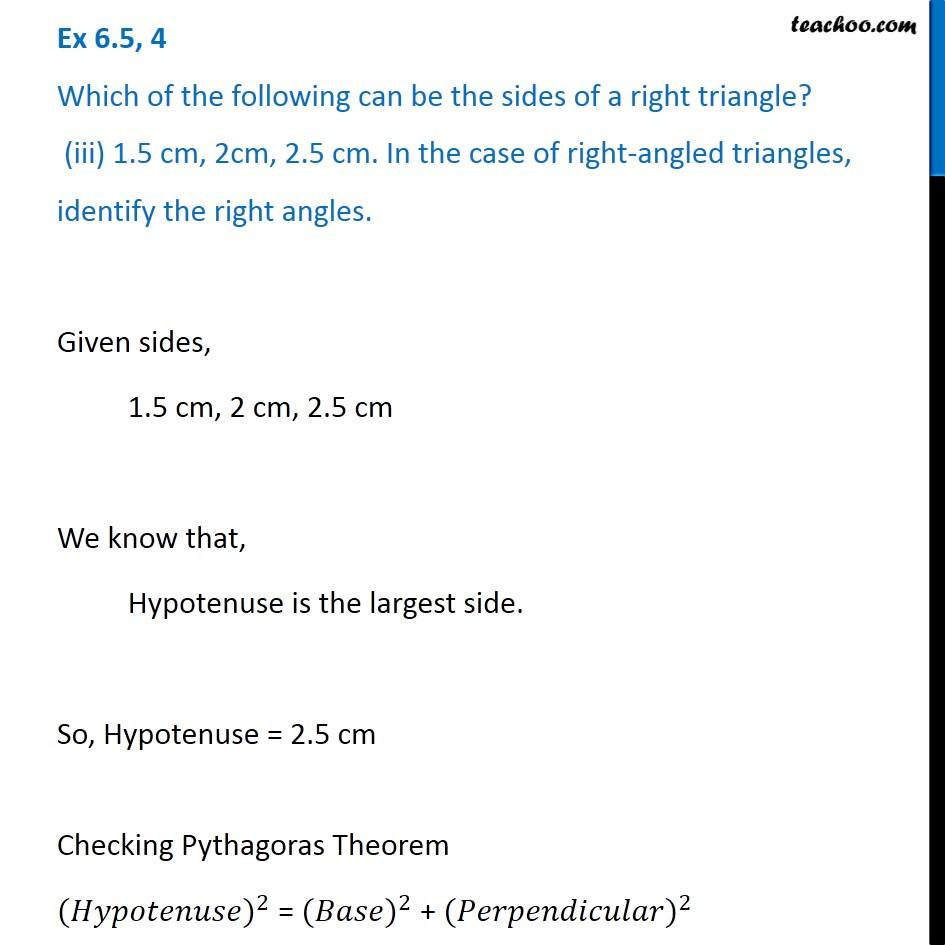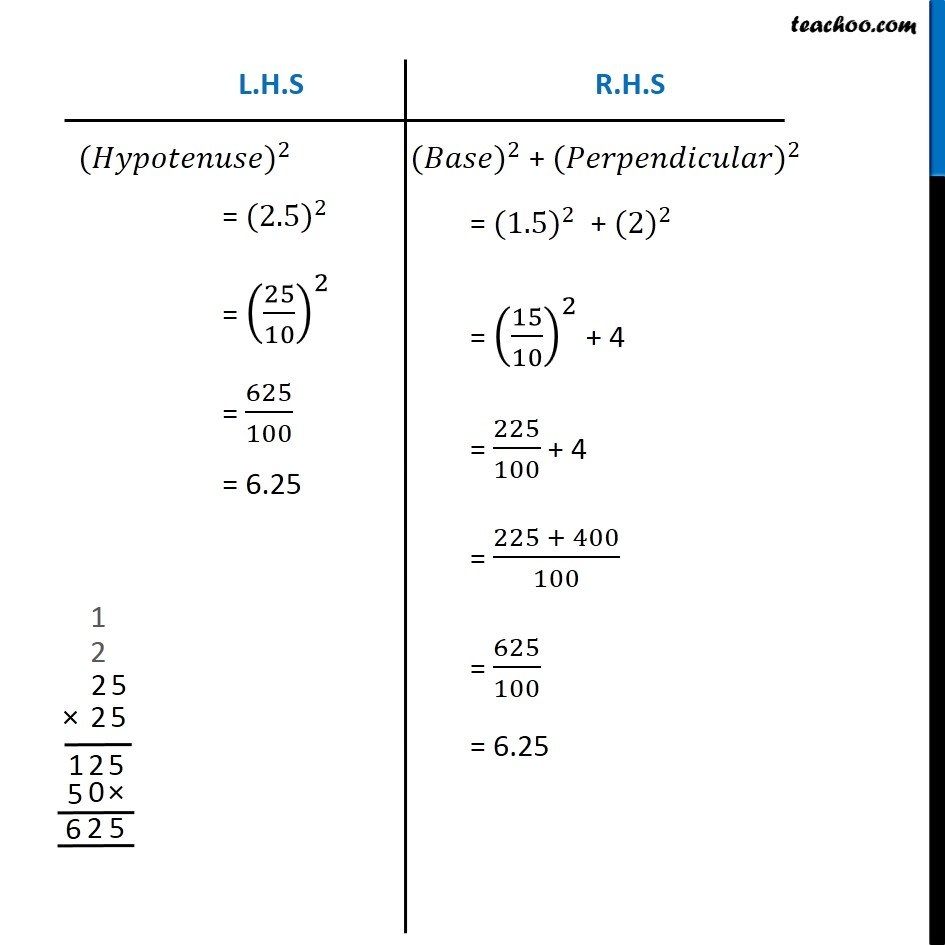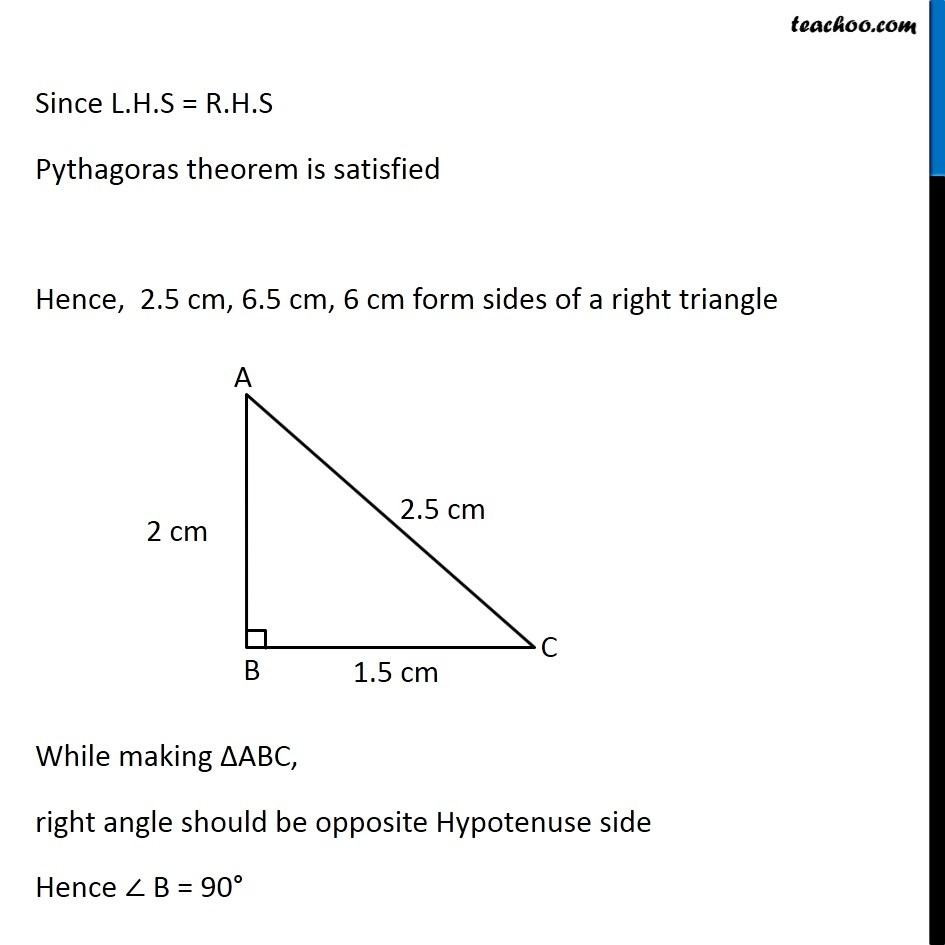Ex 6.5

Chapter 6 Class 7 Triangle and its Properties
Serial order wiseGet live Maths 1-on-1 Classs - Class 6 to 12

### Transcript

Ex 6.5, 4 Which of the following can be the sides of a right triangle? (iii) 1.5 cm, 2cm, 2.5 cm. In the case of right-angled triangles, identify the right angles. Given sides, 1.5 cm, 2 cm, 2.5 cm We know that, Hypotenuse is the largest side. So, Hypotenuse = 2.5 cm Checking Pythagoras Theorem 〖(𝐻𝑦𝑝𝑜𝑡𝑒𝑛𝑢𝑠𝑒)〗^2 = 〖(𝐵𝑎𝑠𝑒)〗^2 + 〖(𝑃𝑒𝑟𝑝𝑒𝑛𝑑𝑖𝑐𝑢𝑙𝑎𝑟)〗^2 〖(𝐵𝑎𝑠𝑒)〗^2 + 〖(𝑃𝑒𝑟𝑝𝑒𝑛𝑑𝑖𝑐𝑢𝑙𝑎𝑟)〗^2 = 〖(1.5)〗^2 + 〖(2)〗^2 = (15/10)^2 + 4 = 225/100 + 4 = (225 + 400)/100 = 625/100 = 6.25 Since L.H.S = R.H.S Pythagoras theorem is satisfied Hence, 2.5 cm, 6.5 cm, 6 cm form sides of a right triangle While making ∆ABC, right angle should be opposite Hypotenuse side Hence ∠ B = 90°